In this post we will use this data in order to explore the QGIS Network Analysis capabilities.

We will focus on the QGIS Processing network analysis tools.

Let’s get started.

The Network Analysis tools are now part of QGIS Algorithms. You can access them by activating the Processing Toolbox.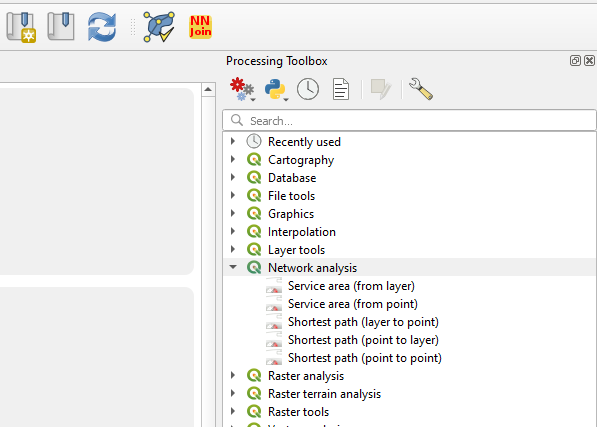These functionalities let you compute least cost paths and service areas (distance or time) taking into consideration your own network data. You need to select the start point and end point, or just the start point (depending on what kind of analysis you are making).

a) Shortest path (point to point)

This algorithm computes optimal (shortest or fastest) route between given start and end points. You need to select the points manually in the map. Like in the image below.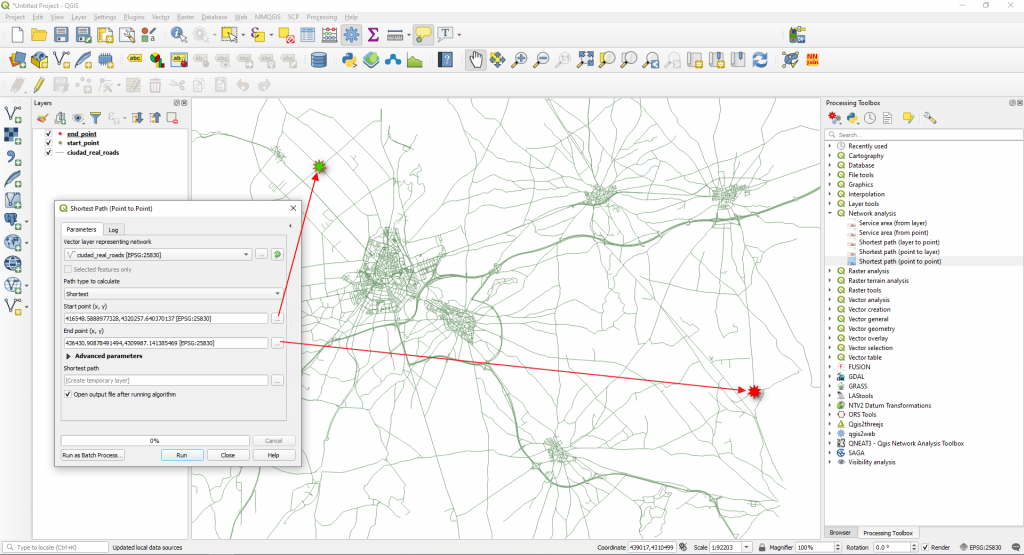We selected the Shortest option in the Path type to calculate. This means that the cost, in this case, represents the distance between the two locations (in layer units). Click Run.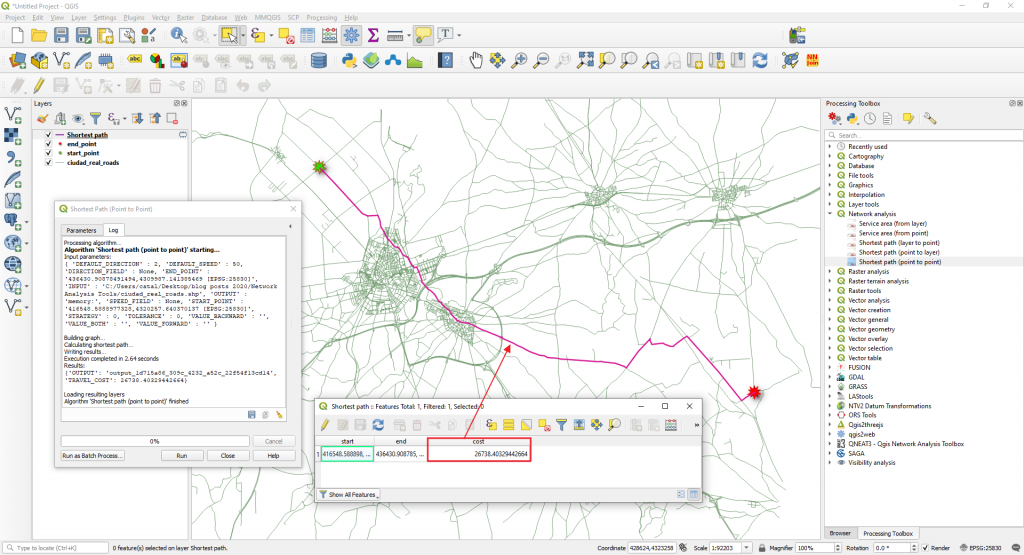A route will be computed (and added as a new layer in the Layers Panel). Between the selected start and end points, the shortest distance is around 26 km.

Next we will go back to the Parameters tab in the Shortest Path (Point to Point) window and we will modify the Path type to calculate option to Fastest.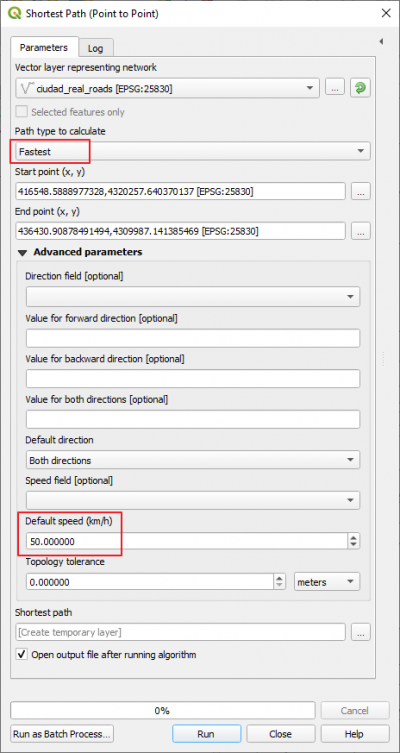The new route will take into consideration the travel speed which is set to 50 km/h and will calculate how much time will take us to go from the start point to the end point (imagine that you are in a car and you can travel with a constant speed of 50 km/h).

A new route, the fastest route will be calculated. You will notice that it overlays with the shortest path (see the blue line route in the image below) but now the cost is time. This means that to get from start point to the end point will take us 0.54 hours.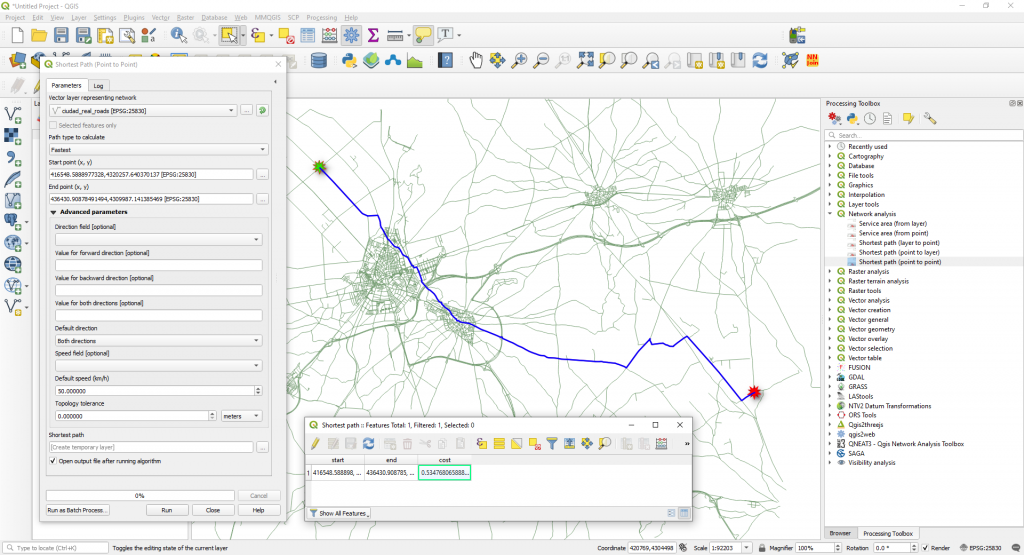You can convert the time cost (which is in hours with fractions) to minutes by multiplying the cost field by 60.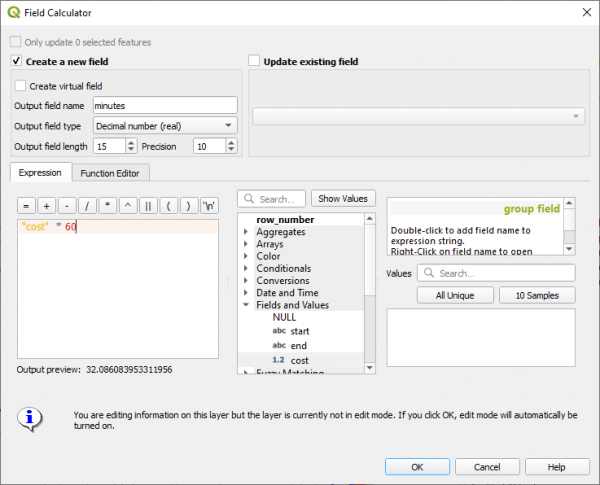The fastest time from the start point to the end point is 32 minutes.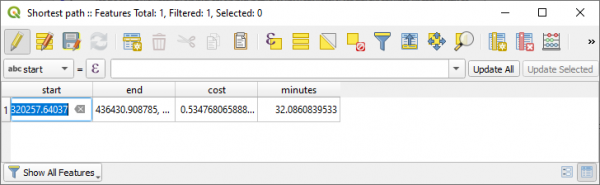NOTE: Take into consideration that we did not take into consideration the road directions. To calculate a route, taking into consideration the road directions you need to fill in the Advanced Parameters available.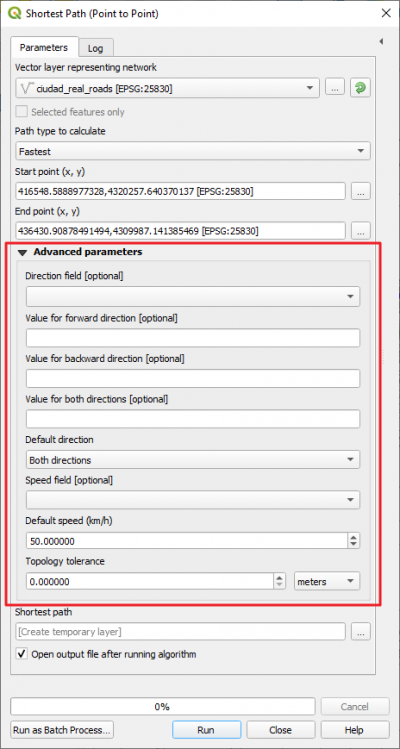In this step you need to have a Direction Field filled in and a Speed Field already calculated.

b) Service Area (from point)

This algorithm creates a new vector with all the edges or parts of edges of a network line layer that can be reached within a distance or a time, starting from a point feature. The distance and the time (both referred to as “travel cost”) must be specified respectively in the network layer units or in seconds.

We will try to identify all the streets that can be reached within 20 minutes from the start point already marked in the map. A 20 minutes service area will show us all accessible streets that can be reached in 20 minutes.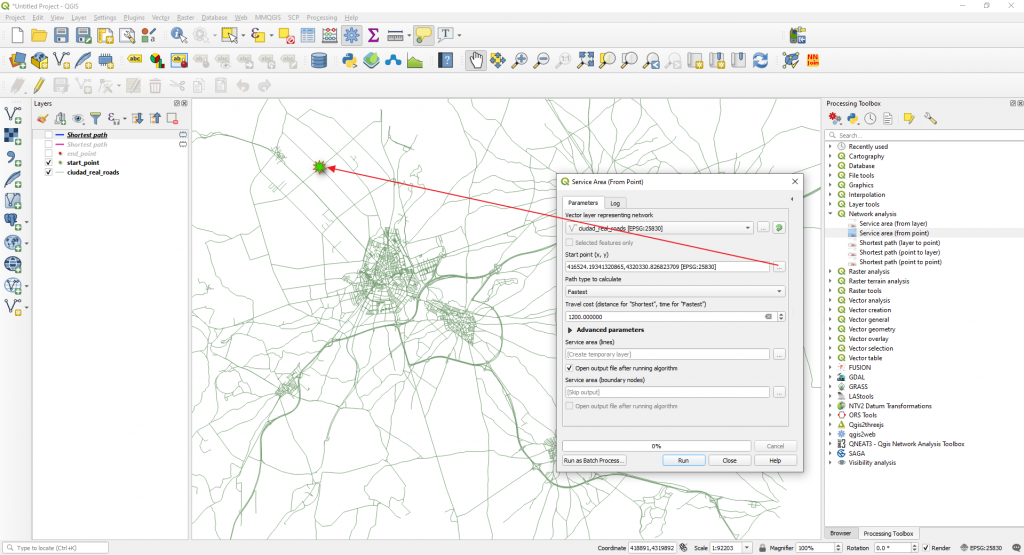We will choose the Fastest option in the Path type to calculate and 1200 seconds (20 minutes) in the Travel cost (distance for “Shortest”, time for “Fastest”.

Make sure you select in the Advanced Parameters in the Default speed (km/h) a value of 30 km/h.

Click Run. The 20 meters service area at a 30 km/h speed is calculated.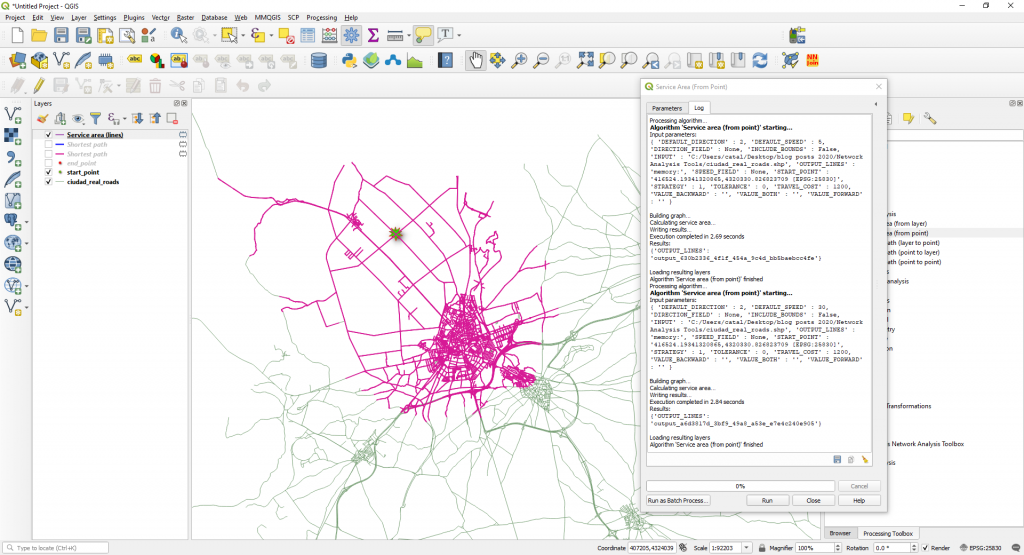NOTE: Take into consideration that we did not take into consideration the road directions.

Quality training taught by professionals